# A Linear Model for Linda

## What is Linda?

Linda is the name of a CrossFit benchmark workout. The original form of the workout is 10-9-8-7-6-5-4-3-2-1 reps of the triplet

• deadlift at 1.5 times bodyweight
• bench press at bodyweight
• clean at 0.75 times bodyweight

Linda was included in the 2018 CrossFit regionals in a standardized form. Based off the average bodyweight of CrossFit games athletes, it was 10-9-8-7-6-5-4-3-2-1 reps of the triplet (weights listed are male/female)

• bench press at 195/135 lb.
• squat clean at 145/105 lb.

Since the weights are fixed, it seems plausible that heavier athletes will finish higher on regionals Linda than lighter athletes. The goal of this post is to make the relationship between bodyweight and Linda finish more precise. To phrase it as a question: if you know an athlete’s bodyweight, how much information does that give you about their Linda finish?

## Data Cleaning

I’m using the same data I used for the visualizations of the regionals, placed in a tibble called regionals:

regionals
## # A tibble: 4,308 x 13
##    rank_overall     id   age weight height name  event  rank points  time
##           <dbl>  <dbl> <dbl>  <dbl>  <dbl> <chr> <dbl> <dbl>  <dbl> <dbl>
##  1            1  55121    25    150   66.5 Katr…     1     1    100 2470.
##  2            2 305891    29    139   63   Kari…     1     4     84 2583.
##  3            3 103389    30    140   65.0 Caro…     1     2     94 2532.
##  4            4   3200    30    148   66   Dani…     1    18     44 2752.
##  5            5 236779    26    146   67   Chlo…     1    24     32 2797.
##  6            6 239148    28    143   63   Caro…     1    12     56 2671.
##  7            7 115892    26    150   66   Meg …     1    14     52 2697.
##  8            8 497688    25    160   67   Kate…     1    17     46 2725.
##  9            9 116926    29    152   67.7 Kari…     1    10     60 2645.
## 10           10 255576    33    155   67   Ashl…     1     5     80 2586.
## # ... with 4,298 more rows, and 3 more variables: cumulative_points <dbl>,
## #   location <chr>, gender <chr>

Let’s look at a summary of the male weights. The weights are self-reported, so there might be some bad data we need to remove. In the process of gathering the data, I converted all weights to pounds (some were originally listed in kilograms).

regionals %>%
filter(gender == "Male") %>%
select(name, weight) %>%
distinct() %>%
select(weight) %>%
summary()
##      weight
##  Min.   :115.0
##  1st Qu.:185.0
##  Median :194.0
##  Mean   :192.7
##  3rd Qu.:200.0
##  Max.   :229.3
##  NA's   :1

The average of 193 lbs is slightly below the average US male weight of 196 lbs. The maximum of about 230 lbs doesn’t sound any alarm bells, but the minimum of 115 lbs is definitely suspicious. After investigating further, this corresponds to an athlete listed as 4 ft. 8 in. in height, which is obviously incorrect, so I will throw out this point. The remaining weights at the lower end seem fine:

regionals %>%
filter(gender == "Male", weight != 115) %>%
select(name, weight) %>%
distinct() %>%
arrange(weight)
## # A tibble: 358 x 2
##    name                 weight
##    <chr>                 <dbl>
##  1 Josh Workman           155
##  2 Rubén Insua            159.
##  3 Ivan Gaitan            161.
##  4 Uriel Bloch            161.
##  5 Gilson Duarte          162
##  6 Alexander Anasagasti   163.
##  7 Tom Lengyel            165
##  8 Aaron Osborne          165.
##  9 Miguel Garduno         167
## 10 ates boran             169
## # ... with 348 more rows

Here is a histogram of the male weight distribution:

regionals %>%
filter(gender == "Male", weight != 115, !is.na(weight)) %>%
select(name, weight) %>%
distinct() %>%
ggplot(aes(weight)) +
geom_histogram(breaks = seq(150, 230, 5), color = "black", fill = "orange") +
scale_x_continuous(breaks = seq(150, 230, 5))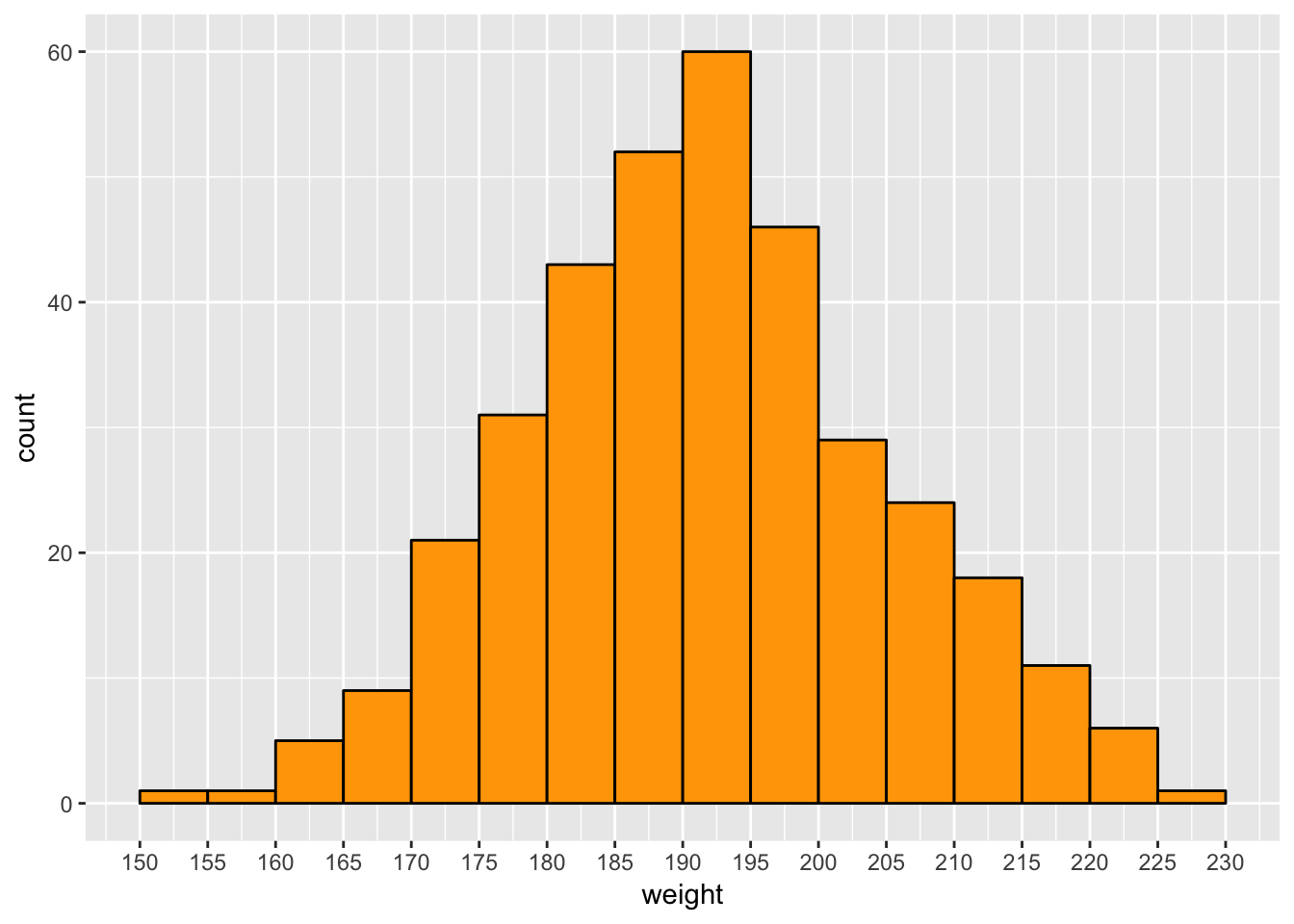For the women,

regionals %>%
filter(gender == "Female") %>%
select(name, weight) %>%
distinct() %>%
select(weight) %>%
summary()
##      weight
##  Min.   :105.0
##  1st Qu.:135.0
##  Median :145.0
##  Mean   :143.7
##  3rd Qu.:150.2
##  Max.   :220.5
##  NA's   :2

Here the average of 144 lbs is significantly below the average US female weight of 169 lbs (this is probably higher now, the 169 lb average is from data from 2014 or earlier: see here). The weight of 105 lbs. comes from someone listed with a height of 4 ft. 10 in., so I will keep this data point. At the upper end, the 220.5 lbs comes from someone listed with a weight of 100 kg and a height of 5 inches, so I’ll throw this out. The next-lowest weight (195 lbs) seems plausible as it comes with a height of about 6 ft. Here is a histogram of the female weight distribution:

regionals %>%
filter(gender == "Female", name != "Steph Dekker", !is.na(weight)) %>%
select(name, weight) %>%
distinct() %>%
ggplot(aes(weight)) +
geom_histogram(breaks = seq(100, 200, 5), color = "black", fill = "orange") +
scale_x_continuous(breaks = seq(100, 200, 5))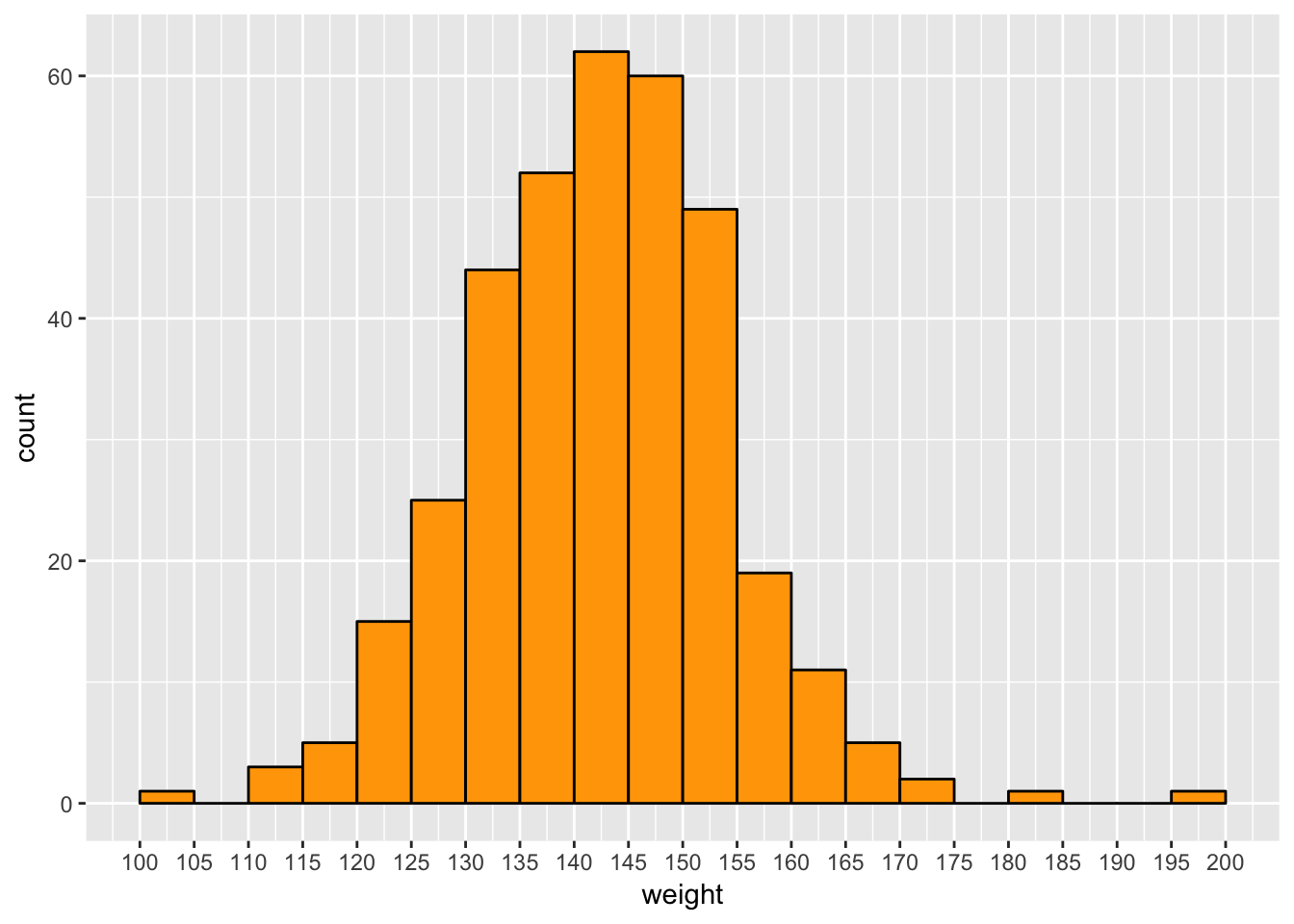## Linda Finish and Bodyweight (Men)

Linda was Event 2 at regionals, so let’s pull out the rows we need. I only need rows with nonzero times, as I used time = 0 to identify people who withdrew from the competition. For people who ran into the timecap of 17 minutes, I used time = -1.

linda_men <- regionals %>%
filter(gender == "Male", weight != 115, !is.na(weight)) %>%
filter(event == 2, time != 0)
linda_men %>%
arrange(rank)
## # A tibble: 353 x 13
##    rank_overall     id   age weight height name  event  rank points  time
##           <dbl>  <dbl> <dbl>  <dbl>  <dbl> <chr> <dbl> <dbl>  <dbl> <dbl>
##  1            9 105536    29   195    69   Chas…     2     1    100  786.
##  2            1 134617    26   195    69   Sean…     2     1    100  879.
##  3            4  92567    34   229.   72.8 Andr…     2     1    100  729.
##  4            1 153604    28   190    67   Math…     2     1    100  761.
##  5           11 105058    28   220    74   Jona…     2     1    100  744.
##  6            3 697349    30   212.   74.0 Andr…     2     1    100  875.
##  7            2 109346    29   195    69   John…     2     1    100  766.
##  8           12 487949    30   207.   70.9 Moha…     2     1    100  841.
##  9            1  16080    27   192.   69.7 Jame…     2     1    100  746.
## 10            1 158264    28   195    71   Patr…     2     2     94  798.
## # ... with 343 more rows, and 3 more variables: cumulative_points <dbl>,
## #   location <chr>, gender <chr>

We can look at the fastest overall times (listed in seconds):

linda_men %>%
filter(time != -1) %>%
select(name, age, weight, height, time, rank, location) %>%
arrange(time)
## # A tibble: 183 x 7
##    name               age weight height  time  rank location
##    <chr>            <dbl>  <dbl>  <dbl> <dbl> <dbl> <chr>
##  1 Andrey Ganin        34   229.   72.8  729.     1 Europe
##  2 Jonathan Gibson     28   220    74    744.     1 West
##  3 James Newbury       27   192.   69.7  746.     1 Pacific
##  4 Mathew Fraser       28   190    67    761.     1 Central
##  5 Adrian Mundwiler    25   183.   67.3  766.     2 Europe
##  6 John Coltey         29   195    69    766.     1 Atlantic
##  7 Roman Khrennikov    23   214.   71.7  771.     3 Europe
##  8 Nicholas Urankar    34   192    70    782.     2 Central
##  9 Gena Malkovskiy     27   216.   70.9  782.     4 Europe
## 10 Chase Smith         29   195    69    786.     1 East
## # ... with 173 more rows

Andrey Ganin in the Europe regional had the fastest overall time of 729 seconds, or 12 minutes and 9 seconds. With a total of 165 reps in this workout, this time corresponds to 1 rep every 4.4 seconds (and there was a lot of walking back and forth between bars)! Europe was a very strong region for this workout - the top 4 finishers in Europe were faster than the first-place finisher in the East.

Here is a plot, along with a linear fit:

linda_men %>%
ggplot(aes(x = weight, y = rank)) +
geom_point(aes(color = location)) +
scale_x_continuous(breaks = seq(150, 230, 10)) +
scale_y_reverse(breaks = c(1:40), minor_breaks = NULL) +
geom_smooth(method = "lm", se = FALSE) +
labs(x = "weight (lbs)", y = "event 2 (Linda) finish")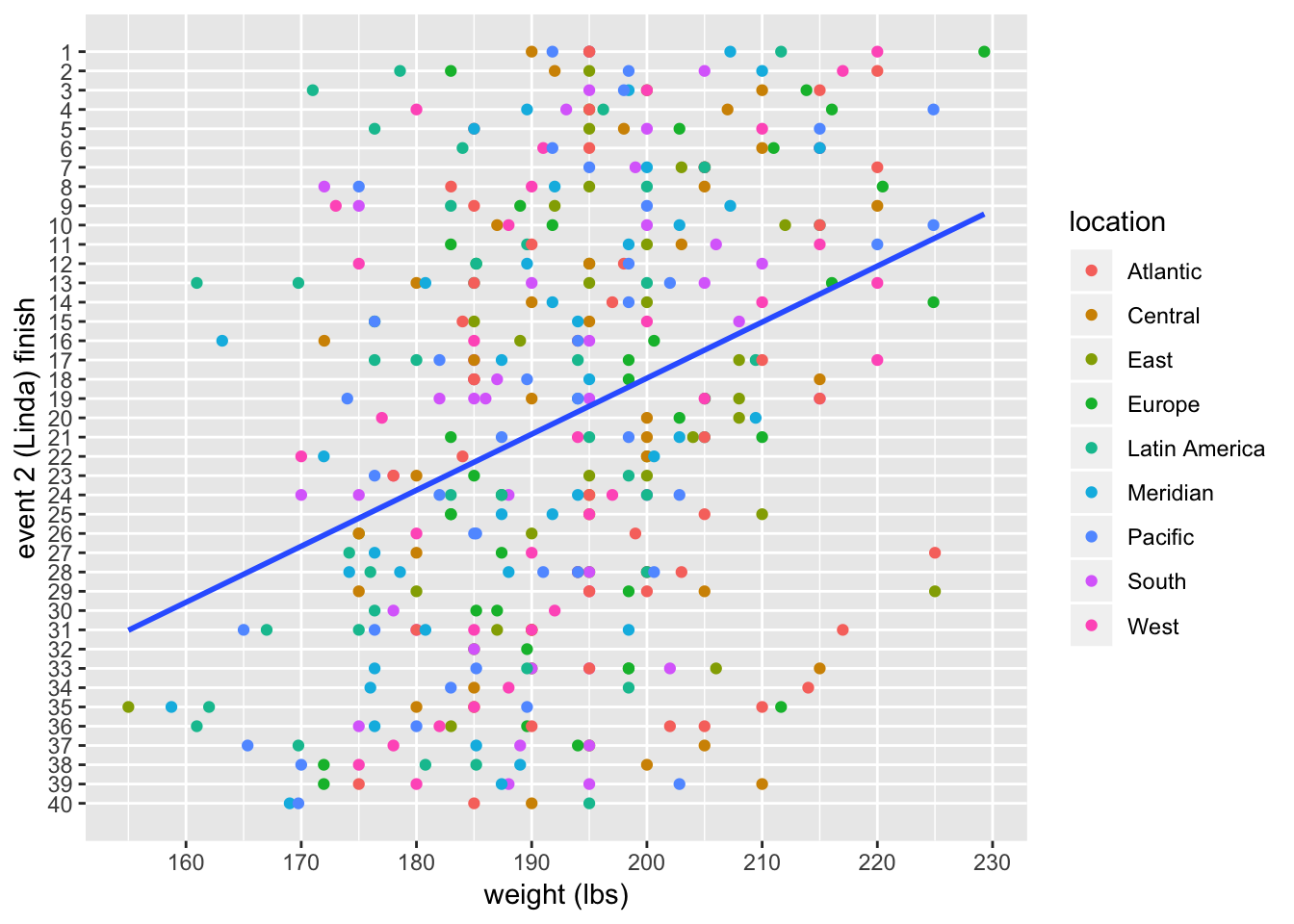Note: I am treating the rank (finish) as a continuous variable, when it is really a discrete variable.

There does seem to be a relationship between weight and finish, but there is a lot of scatter in the plot. Let’s make things more precise by fitting a linear model.

linda_men_model <- lm(rank ~ weight, data = linda_men)
summary(linda_men_model)
##
## Call:
## lm(formula = rank ~ weight, data = linda_men)
##
## Residuals:
##      Min       1Q   Median       3Q      Max
## -23.3706  -8.9394  -0.2724   8.3181  23.9679
##
## Coefficients:
##             Estimate Std. Error t value Pr(>|t|)
## (Intercept) 76.08581    8.02471   9.481  < 2e-16 ***
## weight      -0.29073    0.04149  -7.008 1.25e-11 ***
## ---
## Signif. codes:  0 '***' 0.001 '**' 0.01 '*' 0.05 '.' 0.1 ' ' 1
##
## Residual standard error: 10.63 on 351 degrees of freedom
## Multiple R-squared:  0.1227, Adjusted R-squared:  0.1202
## F-statistic: 49.11 on 1 and 351 DF,  p-value: 1.246e-11

What is this telling us? First, note that the estimated coefficient for weight is negative, but this make sense - higher weight means lower finishing rank. (I flipped the y-axis in the plot above to make it more visually intuitive.) There is a definite association, but the $$R^2$$ is fairly low at 12% and the residual standard error is fairly high at 11 places. This makes sense when looking back at the amount of scatter in the plot.

The coefficient of $$-0.29$$ for weight means that according to the model, the average finish of men weighing 210 lbs is $$0.29(210 - 180) = 8.7$$ places lower than men weighing 180 lbs (lower is better).

Now, there is a problem with using ordinary linear regression here. The outcome variable (event finish or rank) is constrained to be in the interval $$[1, 40]$$. If we apply the model to weights higher or lower than the weights in the data, we will quickly get meaningless results (finishes $$> 40$$ or $< 1$). This is a good reminder that the linear model is only a model and not a very good one. I would expect that weights higher than around 250-260 lbs. would start to correspond to worse finishes: in other words, there should be an optimal bodyweight for this workout, and weights beyond that would not correspond to better finishes. I also expect the relation to fall off faster than a linear function for weights below 160 lbs.

Let’s look at the predictions along with prediction intervals at 95% from the model. This lets us see the large variance in the model.

male_weights = data.frame(weight = c(155:230))
male_predictions <- cbind(male_weights,
predict(linda_men_model, male_weights,
interval = "prediction", level = 0.95))
ggplot(male_predictions, aes(x = weight , y = fit)) +
geom_ribbon(aes(ymin = lwr, ymax = upr), fill = "grey65") +
geom_line(aes(x = weight, y = fit), color = "blue", size = 1) +
geom_point(data = linda_men, aes(x = weight, y = rank)) +
labs(y = "finish") +
scale_y_reverse(breaks = seq(0, 40, by = 10), minor_breaks = NULL)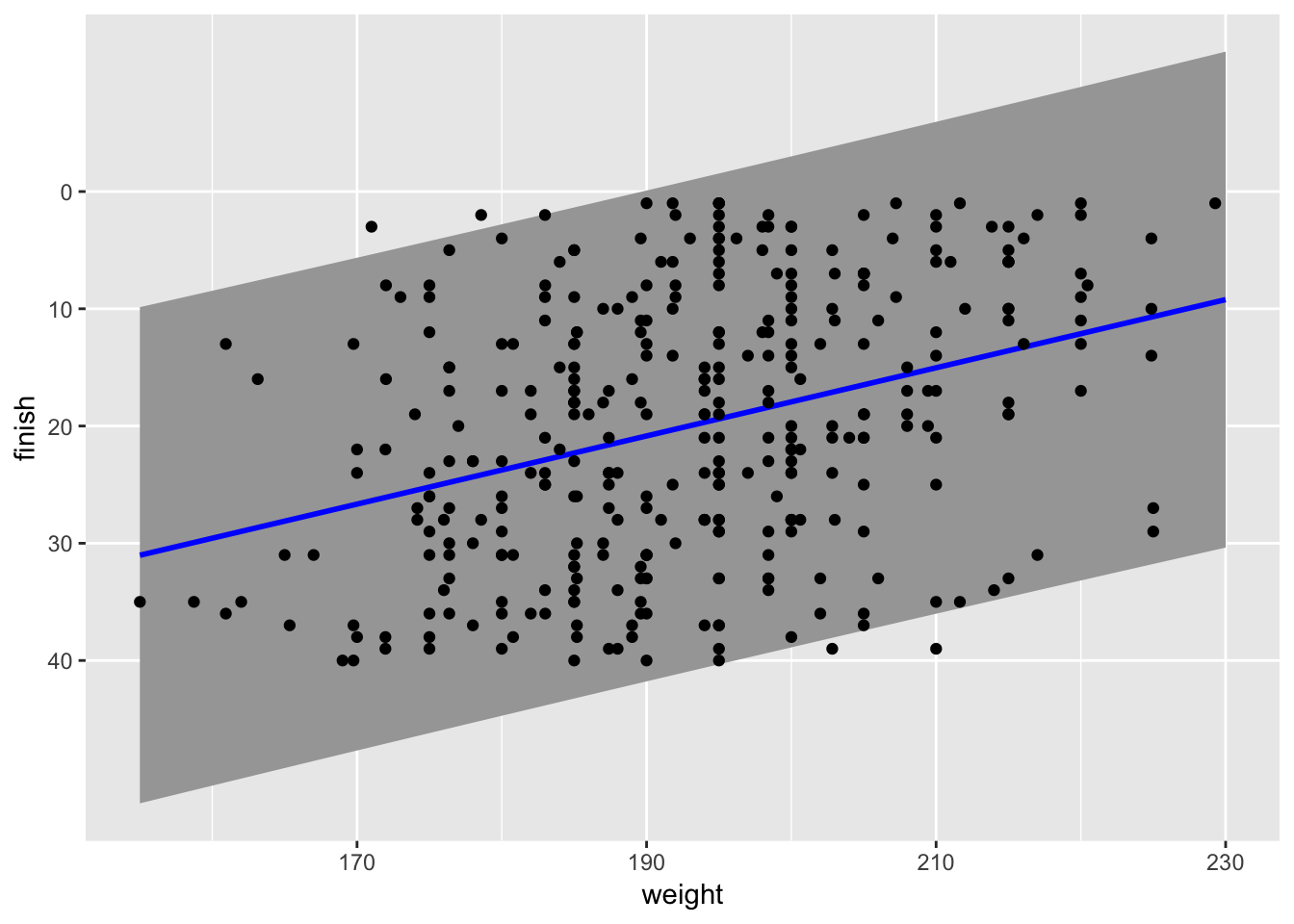## Linda Finish and Bodyweight (Women)

Now for the women,

linda_women <- regionals %>%
filter(gender == "Female", name != "Steph Dekker", !is.na(weight)) %>%
filter(event == 2, time != 0)
linda_women %>%
arrange(rank)
## # A tibble: 353 x 13
##    rank_overall     id   age weight height name  event  rank points  time
##           <dbl>  <dbl> <dbl>  <dbl>  <dbl> <chr> <dbl> <dbl>  <dbl> <dbl>
##  1            1  55121    25   150    66.5 Katr…     2     1    100  770.
##  2           11 208293    27   140    62   Alex…     2     1    100  990.
##  3            3   8859    25   152.   67.3 Ragn…     2     1    100  783.
##  4            1 168305    23   150    66   Broo…     2     1    100  882.
##  5            1 180250    29   157    67   Emil…     2     1    100  860.
##  6           14 239210    32   170    65.0 Andr…     2     1    100  878.
##  7            1 123582    30   140    63   Cass…     2     1    100  834.
##  8            7 274049    31   154.   65.0 Mani…     2     1    100  947.
##  9            1 163097    24   128.   64.2 Tia-…     2     1    100  844.
## 10            3 103389    30   140    65.0 Caro…     2     2     94  866.
## # ... with 343 more rows, and 3 more variables: cumulative_points <dbl>,
## #   location <chr>, gender <chr>

The fastest times for the women:

linda_women %>%
filter(time != -1) %>%
select(name, age, weight, height, time, rank, location) %>%
arrange(time)
## # A tibble: 62 x 7
##    name                          age weight height  time  rank location
##    <chr>                       <dbl>  <dbl>  <dbl> <dbl> <dbl> <chr>
##  1 Katrin Tanja Davidsdottir      25   150    66.5  770.     1 East
##  2 Ragnheiður Sara Sigmundsdo…    25   152.   67.3  783.     1 Europe
##  3 Cassidy Lance-Mcwherter        30   140    63    834.     1 Atlantic
##  4 Whitney Gelin                  33   165    67    834.     2 Atlantic
##  5 Tia-Clair Toomey               24   128.   64.2  844.     1 Pacific
##  6 Emily Abbott                   29   157    67    860.     1 West
##  7 Carol-Ann Reason-Thibault      30   140    65.0  866.     2 East
##  8 Andrea Rodriguez               32   170    65.0  878.     1 Latin Ameri…
##  9 Mekenzie Riley                 30   155    64    880.     3 Atlantic
## 10 Brooke Wells                   23   150    66    882.     1 Central
## # ... with 52 more rows

Katrin Davidsdottir holds the event record, with a time of 12 minutes, 50 seconds. The Atlantic looks like a strong region for this workout: the top 3 Atlantic finishers were faster than the first-place finisher in the Central region.

A plot along with the linear fit:

linda_women %>%
ggplot(aes(x = weight, y = rank)) +
geom_point(aes(color = location)) +
scale_x_continuous(breaks = seq(100, 200, 10)) +
scale_y_reverse(breaks = c(1:40), minor_breaks = NULL) +
geom_smooth(method = "lm", se = FALSE) +
labs(x = "weight (lbs)", y = "event 2 (Linda) finish")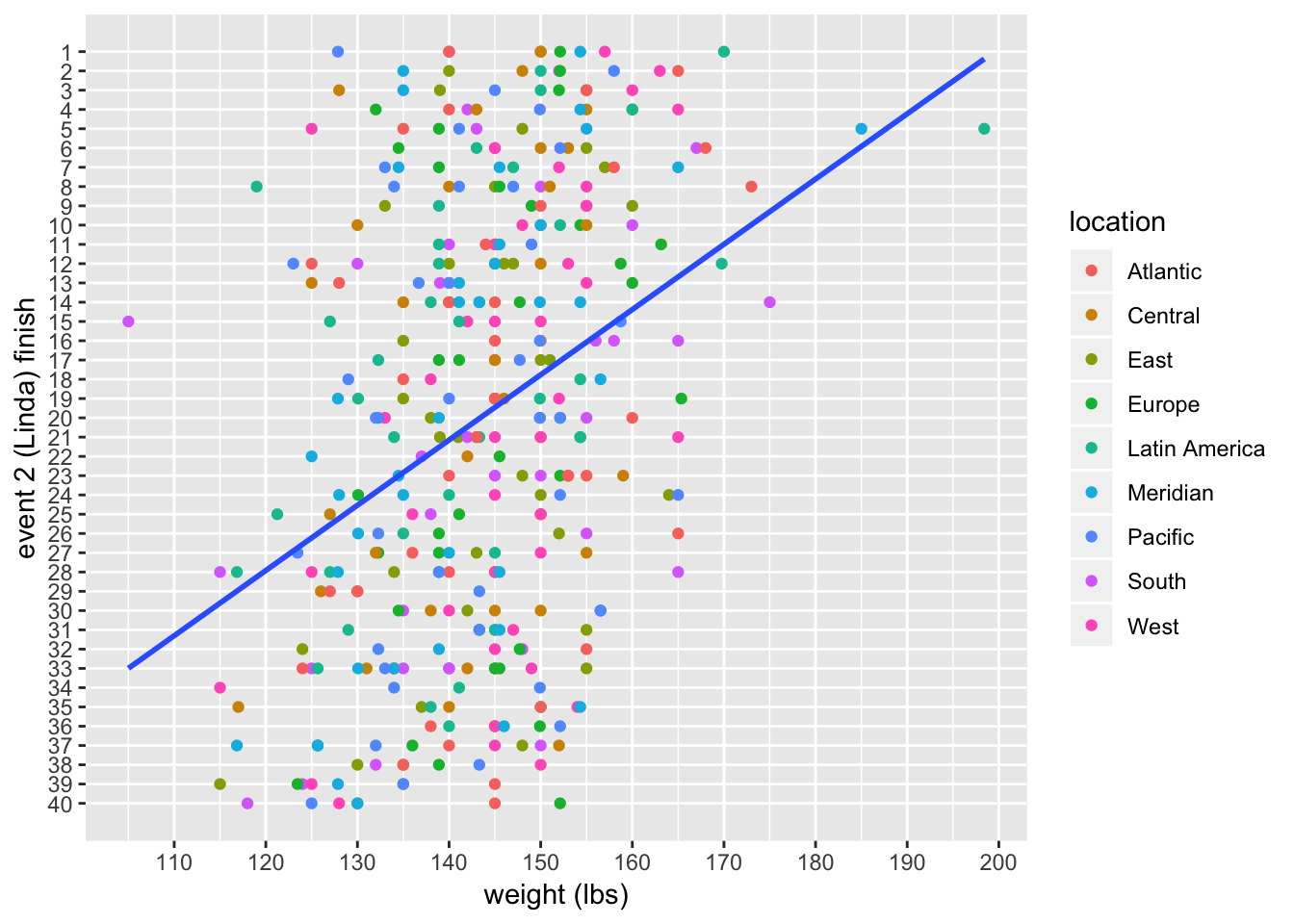There are more outliers here than there were for the men, but again the overall picture looks like a definite relationship, but one with a lot of scatter. Fitting a linear model:

linda_women_model <- lm(rank ~ weight, data = linda_women)
summary(linda_women_model)
##
## Call:
## lm(formula = rank ~ weight, data = linda_women)
##
## Residuals:
##      Min       1Q   Median       3Q      Max
## -24.2562  -8.4573   0.1888   8.7137  22.9523
##
## Coefficients:
##             Estimate Std. Error t value Pr(>|t|)
## (Intercept) 68.53752    6.90259   9.929  < 2e-16 ***
## weight      -0.33848    0.04794  -7.060 8.96e-12 ***
## ---
## Signif. codes:  0 '***' 0.001 '**' 0.01 '*' 0.05 '.' 0.1 ' ' 1
##
## Residual standard error: 10.74 on 351 degrees of freedom
## Multiple R-squared:  0.1244, Adjusted R-squared:  0.1219
## F-statistic: 49.85 on 1 and 351 DF,  p-value: 8.96e-12

The $$R^2$$ value and the standard error are almost exactly the same as the model for the men. The coefficent for weight has a larger magnitude: $$-0.34$$ for the women comparted to $$-0.29$$ for the men. According to the model, the average finish of women at 165 lbs is $$0.34(165-135) = 10.5$$ places lower than the average finish of women at 135 lbs (remember that lower is better).

The predictions along with 95% prediction errors:

female_weights = data.frame(weight = c(100:200))
female_predictions <- cbind(female_weights,
predict(linda_women_model, female_weights,
interval = "prediction", level = 0.95))
ggplot(female_predictions, aes(x = weight , y = fit)) +
geom_ribbon(aes(ymin = lwr, ymax = upr), fill = "grey65") +
geom_line(aes(x = weight, y = fit), color = "blue", size = 1) +
geom_point(data = linda_women, aes(x = weight, y = rank)) +
labs(y = "finish") +
scale_y_reverse(breaks = seq(0, 40, by = 10), minor_breaks = NULL)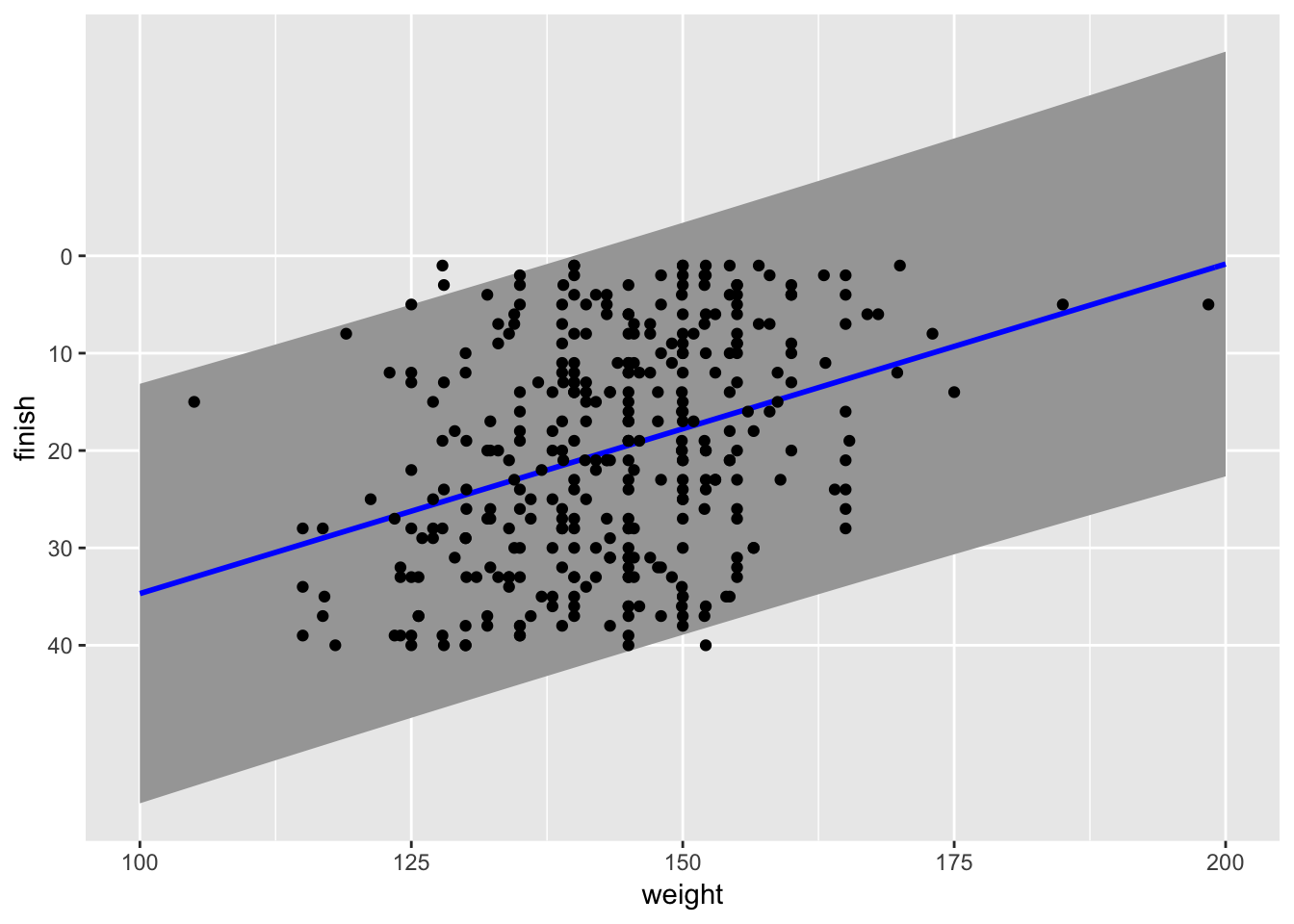##### Landon Lehman
###### Data Scientist

My research interests include data science, statistics, physics, and applied math.